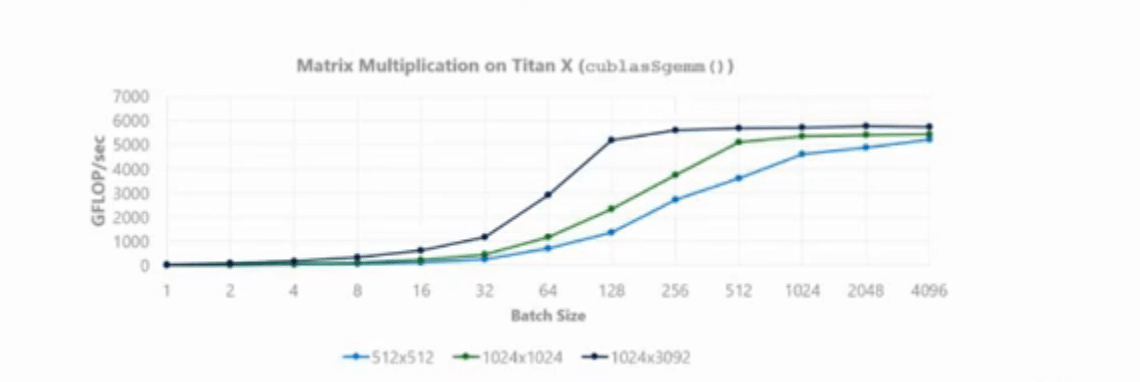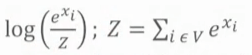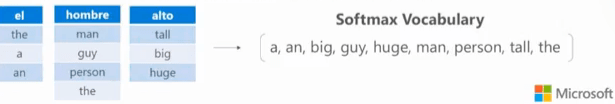# A Practical Guide to Neural Machine Translation

This talk focuses on engineer techniques for large-scale NMT systems. It helps us to understand how GPU works and how to make machine translation faster with the help of GPU.

For example: how do engineers bring the training time for a state-of-the-art NMT system down from 15 days to 3 days on a single GPU.

### Highlight Review

• A Brief History of Neural Machine Translation
• 【2003】 Large-scale neural networks for NLP started with Yoshua Bengio’s feed-forward neural language model
• 【2014】 Sequence-to-Sequence (Sutskever et al) processes source sentence with RNN, gets a hidden state, and uses it to run another RNN language model reproduce one word each time step on the target side
• 【2015】 Attention model (Bahdanau et al), which is the basis of most current NMT implementation
• A common problem of all aforementioned methods is that training takes a long time (several weeks with multiple GPUs)
• The training time is painful, and it is very hard to do research with such long turn around time.
• RNN structures and GPU optimization background
• Let’s take a look of the structure of RNN• The element-wise functions are implemented as Cuda kernels, so that same kernel code is executed on many Cuda cores in parallel.
• The larger batch sizes you have, the more efficiency you get (matrix multiplication with small batch sizes are very inefficient). Because larger matrix can make better use of GPU cores and RAM• The problem is that we cannot simply use a large batch size (for example 1024)
• For RNN, a mini-batch is a collection of sequences
• Large batch of sequences probably won’t be able to fit into memory, and will cause training inefficiencies
• How do we optimize RNN with GPU?
• GEMM fusion: combine all matrix multiplications into a single call
• one type of GEMM fusion is to fuse all weight matrixes together (fuse w)
• another type of GEMM fusion is fuse across time step (fuse x_i,…,x_n)
• if combine weights fusion and time steps fusion, we get better efficiency
• Element-wise fusion: combine all element-wise functions
•• fuse all element-wise function into a single kernel, which would be run the element-wise functions in sequence
• Variable-length sequences
• pad all sequences to the maximum size (very inefficient)
• terminate the recurrent loop early
• Use buckets, i.e. different bucket can has different network graphs with different maximum sequence lengths
• Bucketing neural network
• different buckets can have different batch sizes. Just need to make sure total words number is fixed. i.e. batch size = 4000 words, bucket [7,11] has 384 sentences, bucket [40,50] has 64 sentences, notices that the sentences number is better round to multiple of 64
• fixed batch size make sure the magnitude of gradients are similar, thus training is more stable
• Large output vocabulary
• A typical vocabulary size for NMT is 100,000; A typical hidden layer size is 1024. This means the output layer is an [n * 1024 * 100000] GEMM, where n is the batch size. Computing the full softmax every time is painful. One solution is that we can sub-sample the output layer, and compute part of the softmax.
• The best sub-sampling method that can be implemented with GPU is Importance Sampling (Bengio & Senecal, 2008); Other sub-sampling methods: Noise Contrastive Estimation, BlackOut layer, Hierarchical Softmax.
• Importance Sampling.
• The softmax function convert logit x_i to probabilities over all words in the vocabulary. Importance sampling aims to approximate Z using a portion of the vocabulary.
•• The importance sampling formulation: Always include top m most common words, n random words from the vocabulary. Sampled vocabulary shared across minibatch
• Approximate softmax function is shown below, notice that we need to multiply the randomly sampled n words’ scores after exponentiated them by a factor of α, α = (|V| – m ) / n
•• Why multiply α? If we dont do this, rare words will have inflated probabilities. i.e. We have 100,000 size of vocabulary, we first get the top m=8,000 most common words. Then randomly sample n = 8,000 words from 92,000 remaining words, which means each words in the random sample has been sampled 92,000/8,000 ≈ 11.5 times. Multiplying α to the rare word sample makes estimated Z bigger, also push down the probability of rare word.
• The following table shows the loss, test set BLEU score, and training time for full softmax and sampled softmax
•• Training on multiple GPUs
• Train on 2-4 GPUs using asynchronous SGD. Each GPU processes a different minibatch in parallel, updates a set of “master” weights independently of one another
• The gradients/weights must be transferred from the GPU to/from the parameter server (GPU or CPU)
• Inference(testing)
• Difference between training and inference: Training is on GPU, inference is on CPU or GPU; Training uses large batch, inference uses small batch; During training weights change every batch, at inference they are fixed; At training time, the source and target networks are equally expansive, at inference time, the target network is b times of the source network (b associated with the beam search window size)
• large vocabulary problem: computing the full softmax over 100,000 words is intractable, we wanna the test time is much shorter than training time. Therefore, we prune the vocabulary size to a few hundred words which are plausible for the current sentence. We usually run a standard word alignment (“IBM Alignment”) to produce a lookup table. (blue cells have the source words, light blue cells are the lookup tables for each source words, combine all words in the lookup table and get the shortlist vocabulary that really goes into softmax)
•• Efficient matrix multiplication on CPU
• small batch size (1 to 8)
• Intel MKL and OpenBLAS have very optimized floating point matrix multiplication implementations
• Model selection:
• The target is much more expansive than source (because of beam search)
• The first layer is 1/2 the cost of all other layers (because of pre-computation)
• The cost of a layer is quadratic with the size ( i.e. 1024-dim is 4 times the cost of 512-dim）
• GRU is 25% cheaper than LSTM
• Model ensemble can be worthwhile, but has diminishing returns

### Questions:

• In importance sampling, why multiply α to the randomly sampled n words?  What will happen if we don’t do that?
• How to choose m and n? Empirically?

Original article from Synced China http://www.jiqizhixin.com/ | Localized by Synced Global Team | Author: Yuting Gui